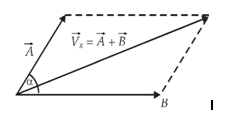Courses

# Addition and Subtraction of Cartesian Vectors Mechanical Engineering Notes | EduRev

## Mechanical Engineering : Addition and Subtraction of Cartesian Vectors Mechanical Engineering Notes | EduRev

The document Addition and Subtraction of Cartesian Vectors Mechanical Engineering Notes | EduRev is a part of the Mechanical Engineering Course Engineering Mechanics - Notes, Videos, MCQs & PPTs.
All you need of Mechanical Engineering at this link: Mechanical Engineering

Addition of Forces. The concept of force resultant can be applied to a concurrent force system which is written in the Cartesian vector form.  If P is the resultant of F1, F2, F3, … hence

PFi

=  (F1x + F2x + …)i + (F1y + F2y + …)j + (F1z + F2z + …)k

= ΣFixi + ΣFiyj + ΣFizk          (2.5)

whereΣFix, ΣFiy, and ΣFiz are the sums of the magnitudes of the forces in the corresponding direction.

2.8 RESOLUTION OF FORCES

Any force can be resolved into its components. There are a number of methods that can be used to resolve a force. The method use depends on the problem at hand.  The different methods are described below.

2.8.1. Parallelogram Law

A force acting at any point can be resolved into components that act in two desired directions through the parallelogram law.  Force F in Figure 2.12(a), for example, can be replaced by two components acting in directions 1 and 2.  The resolution is implemented by drawing a parallelogram with F as the diagonal and its two non-parallel sides along directions 1 and 2,Note that resolution is the reverse process of adding two forces into their resultant.  Hence the resolution involves six quantities, i.e. the magnitude and direction of F and of the two components, where two of them can be determined when the other four are known.

Rectangular components.  When force F is resolved into perpendicular directions, we obtain the rectangular components.  This is shown in Figure 2.13 for a 2D case, where the respective perpendicular directions are represented by the x-axis and the y-axis.  In this case, we get

Fx = F cos θ

Fy = F sin θ

where  θ = tan-1 (Fy/Fx)

Addition Of Forces.   Forces can be added by using their components, normally the rectangular components in the Cartesian directions. Consider forces F1 and F2 that are concurrent at O being added using the force polygon, Figure 2.14(a).  The resultant P is

P = F1 + F

Offer running on EduRev: Apply code STAYHOME200 to get INR 200 off on our premium plan EduRev Infinity!

,

,

,

,

,

,

,

,

,

,

,

,

,

,

,

,

,

,

,

,

,

;